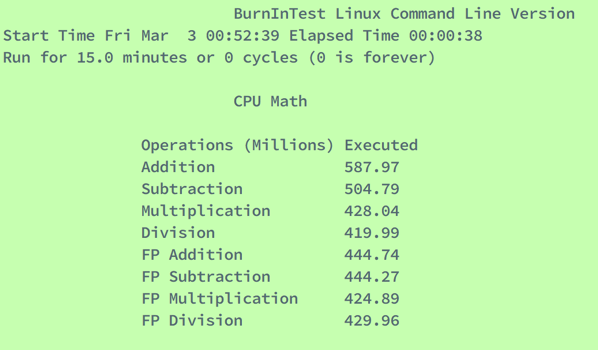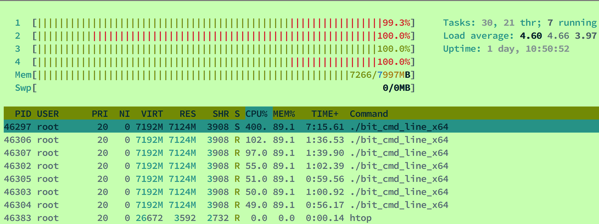### BurnlnTest

`wget http://www.passmark.com/ftp/burnintest_2.1.tar.gz`

```#!/bin/bash

#Check if 64 bit or 32 bit
platform=\$(uname -m)
if [ "\$platform" = "x86_64" ]; then
bitexe="./64bit/bit_gui_x64"
echo "64-bit OS detected, launching" \$bitexe
else
bitexe="./32bit/bit_gui_x32"
echo "32-bit OS detected, launching" \$bitexe
fi

echo
#Check if required libraries are there, warn if not

#for i in \$( ldd \$bitexe |cut -f2 | cut -d' ' -f1 ); do
count=0
for i in \$( ldd \$bitexe | grep "not found" |cut -f2 | cut -d' ' -f1 ); do
echo "Missing Library: " \$i
count=1
done

echo
if [ \$count -gt 0 ]; then
echo "Missing libraries detected!"
echo "To use the GUI version of BurnInTest you will need to install the above"
echo "libraries using your prefered package manager before running BurnInTest"
echo "A command line version of BurnInTest that requires less libraries"
echo "is also available in the 32bit and 64bit directories."
else
exec \$bitexe
fi```

`./bit_cmd_line_x64`

```BurnInTest Linux Command Line Version
Start Time Fri Mar  3 00:48:33 Elapsed Time 00:00:21
Run for 15.0 minutes or 0 cycles (0 is forever)

Test Name                Cycle  Operations       Errors Last Error Description
CPU - Maths              20     1.953 Billion    0      No error
Memory (RAM)             0      11.336 Billion   0      No error
Network: 127.0.0.1       34628  304 Million      0      No error

Use ESC or CTRL-C to exit. Use number keys to switch to single test screen;
1: Main Menu     2: CPU Math Test 3: Memory Test   4: Disk Test   5: DVD/CD Test
6: Network Test  7: Serial Test   8: Parallel Test 9: USB Test``````CPU Maths Test
The CPU test exercises and verifies a wide range of CPU instructions, at user specified loads. The user selectable sub-categories of the CPU test are:
• General purpose instructions (x86)
• Floating Point Unit (FPU) instructions (x87)
• CPU extension instructions (x86 extensions). The specific extension instructions may be selected: SSE, SSE2. • Prime number test
The numbers displayed in the test window for this test represent how many millions of CPU operations have been performed and verified. Each different math’s test is run for half a second. After all tests have been run the cycle count is incremented. The duty cycle and the CPU speed determine how many operations can be processed during the half second period. By default, a CPU test is run on each CPU core.
General purpose instructions
This test exercises and verifies correct operation of CPU instructions from the following groups:
· Integer mathematics (e.g. add, subtract, multiply and divide)
· Data transfer instructions (e.g. pushing/popping data to/from the stack) · Bitwise logical instructions (e.g. bitwise and/or/xor)
· Shift and rotate instructions (e.g. shift data left x number of bits)
· Logical instructions (e.g. equals)
· Control transfer instructions (e.g. jump on x greater than y)
· String instructions (eg. copy a text string)
Known and random data sets are used to exercise and verify correct operation. The random numbers are generated regularly to provide a larger data set, as well as ensure that the CPU caches overflow and that this mechanism is tested.
Floating Point Unit (FPU) instructions
This test exercises and verifies correct operation of CPU instructions from the following groups: · Floating Point math (e.g. add, subtract, multiply and divide)

· Transcendental's (e.g. sin, cos)
Extension instructions (x86 extensions)
This test exercises extensions to the x86 CPU instruction set for a variety of applications, such as multimedia.
SSE : As above, but for SSE instructions. Instructions include moving SSE registers, adding, subtracting, and multiplying. Data set: groups of 4 x 32-bit floats (e.g. a,b,c,d) where a,b,c and d are 32-bit floats.
SSE2: As for the SSE test, but for SSE2 instructions. Data set: pairs of 64-bit floats (e.g. x,y) where x,y are 64-bit floats.
Prime number
This test exercises and verifies correct operation of CPU through the use of a prime number generation algorithm.
[your_command_line_prompt]# cat /proc/cpuinfo
This command will show your CPU info, including speed, cache size and features.```

`http://www.passmark.com/ftp/BIT_Linux_Help.pdf``Run for 15.0 minutes or 0 cycles (0 is forever)`

`Run for 1.0 minutes or 0 cycles (0 is forever)`

-X 1的结果：

`Run for 15.0 minutes or 1 cycles (0 is forever)`